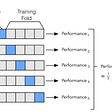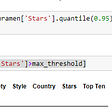# Working with seaborn Python library: visualizing foot traffic based on big data of MAC addresses

1. Split the time code to know hourly data. For example, since the first number of string ‘01:23:45’ split by colon delimiter would give ‘01’, any row of data where time field has starting with ‘01’ would be data from 1 am.
2. Add MAC addresses to an array as the script runs through.
3. Then, once the hour section of time changes, count number of elements in MAC addresses array, and map this number to the past hour in a dictionary.
4. Empty the MAC addresses array.
5. Repeat.
`import numpy as npimport seaborn as snsimport pandas as pdimport netaddrfrom matplotlib import pyplot as pltimport csvimport retimes = []uniqueMACcount = []currentcount = '0'uniqueMAC = []#convert the timesdef split_by_hour(h):    h = re.split('[:]', h)    return hwith open("seaborn.csv") as f:    reader = csv.reader(f)    next(reader) # skip header    data = [r for r in reader]for element in data:    if currentcount == split_by_hour(element):            if element not in uniqueMAC:                try:                    if (netaddr.EUI(element).words & 0b10) == 0 and (netaddr.EUI(element).words & 0b01) == 0:                        uniqueMAC.append(element)                except (netaddr.core.AddrFormatError):                    pass            if currentcount != split_by_hour(element):        times.append(currentcount)        uniqueMACcount.append(len(uniqueMAC))        currentcount = split_by_hour(element)        uniqueMAC = []    times.append('19')uniqueMACcount.append(len(uniqueMAC))times = list(map(int, times))df = pd.DataFrame(dict(hour=times, count=uniqueMACcount))print(df.head(15))sns_plot = sns.factorplot("hour","count", data=df,kind="bar",size=6,aspect=2,legend_out=False)sns_plot.savefig("output.png")`

--

--

--

## More from stine

r&d blog on architecture, software engineering and inspirations

Love podcasts or audiobooks? Learn on the go with our new app.

## Task 2: Automated Web Development and Testing using Jenkins## Design Problems with Major Software## Solving a Rubik’s Cube Using Graph Theory## Android: Continuous Integration with Travis CI## CS 371g Summer 2020: Yin Deng## LeetCode #1365 “How Many Numbers Are Smaller Than the Current Number”## stine

r&d blog on architecture, software engineering and inspirations

## Working of k-fold cross validation## Employees Attrition Analysis — #DS001## Dealing with outliers in dataset(Python).## An introduction to Meaning of Data